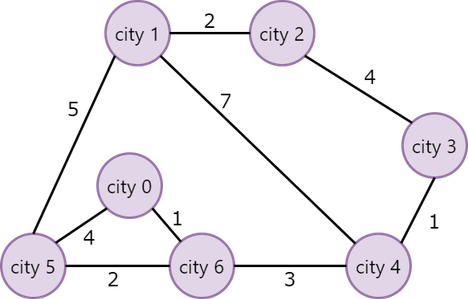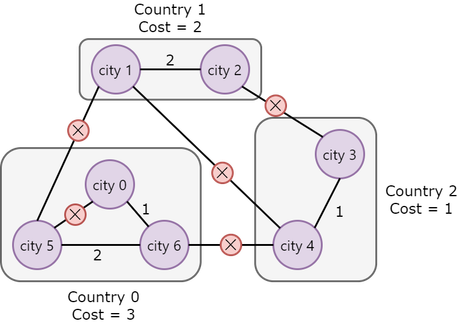# Efficient Construction on Marsakash_sustReplay of Cefalo SUST Int...
Limits 1s, 512 MB

Elon Musk has constructed $N$ cities numbered from $0$ to $N - 1$ on Mars. He initially planned to construct $E$ bidirectional roads to connect different cities (where the construction cost of the $i$th road is $W_i$. One such example for $N = 7$ and $E = 9$ can be seen below:In the figure, the numbers near each road are the cost of constructing that road.

However, Mr. Musk changed his mind a bit. Now, he wants to establish $C$ countries numbered from $0$ to $C-1$. Then, he will distribute all the cities among these countries following a special rule. After the distribution, Mr. Musk wants to construct some or all of the $E$ roads he planned initially in such a way that every pair of cities in each country are connected either directly via a road or through another city. Cities from different countries need not be connected. Mr. Musk will distribute the cities so that the cost of constructing such roads is minimized. One such construction of the example shown above can be seen below (for $C = 3$):Note that the roads marked with a $\times$ symbol are not constructed. Here, Mr. Musk constructed $3$ countries in such a way that the cost of building roads for each country is minimized. The cumulative costs of building roads for countries $0$, $1$, and $2$ are $3$, $2$, and $1$, respectively.

Now, Mr. Mask has asked you to determine the maximum amount among the cumulative costs of building roads following the rules specified above. In the given example, the maximum cumulative cost is $3$.

Given the configuration of the cities and the number of countries, you need to print the maximum cumulative cost. If such construction is not possible, you need to print $\text{-}1$.

It is to be noted that it is possible that a country does not have any city in it.

## Input

The first line contains three space-separated integers $N \, (1 \leq N \leq 14)$, $C \, (1 \leq C \leq 14)$and $E \, \left(0 \leq E \leq \frac{N\times (N - 1)}{2}\right)$ which denote the number of cities, countries, and roads, respectively.

Then $E$ bidirectional roads will be given in the form of three space-separated integers: $U_i$, $V_i \, (0 \leq U_i, V_i \leq N - 1)$ and $W_i \, (1 \leq W_i \leq 10^8)$ where $U_i$ and $V_i$ denote the cities at the end of the road and $W_i$ denotes its weight.

## Output

Print the maximum among the minimized cumulative costs. If there is no valid answer, print $\text{-}1$.

## Sample

InputOutput
7 3 9
1 2 2
1 5 5
1 4 7
2 3 4
3 4 1
4 6 3
0 5 4
0 6 1
5 6 2

3### Submit